Online Tuning of Power System Stabilizers using Fuzzy Logic Network with Fuzzy C-Means Clustering | OMICS International
Journal of Electrical & Electronic Systems

# Online Tuning of Power System Stabilizers using Fuzzy Logic Network with Fuzzy C-Means Clustering

Hajizade Kanafgorabi M* and Dr. Karami A

Department of Electrical Engineering, Faculty of Engineering, University of Guilan, Rasht, Iran

*Corresponding Author:
Electrical Engineering Department
Faculty of Engineering
University of Guilan
Rasht, P.O. Box 3756,Iran
Tel: +98-131-6690274
E-mail: [email protected]

Received Date: September 10, 2014; Accepted Date: December 30, 2014; Published Date: January 15, 2015

Citation: Hajizade Kanafgorabi M, Karami A (2015) Online Tuning of Power System Stabilizers using Fuzzy Logic Network with Fuzzy C-Means Clustering. J Electr Electron Syst 4:136. doi:10.4172/2332-0796.1000136

Copyright: © 2015 Hajizade Kanafgorabi M, et al. This is an open-access article distributed under the terms of the Creative Commons Attribution License, which permits unrestricted use, distribution, and reproduction in any medium, provided the original author and source are credited.

Visit for more related articles at Journal of Electrical & Electronic Systems

#### Abstract

Power system stabilizers (PSS) have been widely used to enhance damping due to the electromechanical low frequency oscillations occurrence in power systems. In this paper, a new method is used for the online tuning of parameters of conventional power system stabilizers (CPSS) using fuzzy logic. Fuzzy logic enables mathematical modeling and computation of some nonlinear parameters of the system, which are usually, derived empirically by utilization of expert knowledge rules. Various literatures has shown that fuzzy logic controller is one of the most useful methods for expert knowledge utilization. This type of controller is adaptive in nature and can be used successfully as a power system stabilizer. The design of fuzzy logic controllers is mainly based on fuzzy rules and input/output membership functions. Simple and efficient clustering algorithms allow data classification in distinct groups using distance and/or similarity functions. In the present paper, the optimum generation of fuzzy rules base using Fuzzy C-means (FCM) clustering technique is used. In fact, data are classified and the number of fuzzy rules which depends on convergence radius is determined. Finally, the performance of proposed FCM controller is compared with that of conventional controller. The active power, reactive power and bus voltages used as inputs to the fuzzy logic network based power system stabilizer and the parameters of the optimum stabilizer , i.e. gain factor as well as time constants of the lead/lag compensator, are
the outputs of the proposed system. The design method has been successfully implemented on a single machine power system connected to an infinite bus over various operating conditions.

#### Keywords

Dynamic stabilizer; Power system stabilizers; online tuning of parameters; Fuzzy C-Means clustering Prediction

#### Introduction

Power systems are complex and nonlinear. In these systems, electromechanical low frequency oscillations are produced. The electromechanical oscillations between connected synchronous generators are an inherent phenomenon. To overcome these oscillations, power system stabilizer is widely used. Recent research is related to lead/lag compensators [1-3].

Electromechanical low frequency oscillations in transmission networks are an important issue in power systems which its study will contribute largely to stability problem [1,2]. The performance of excitation systems and high gain AVR in terms of transient stability improvement and normal performance of the system is very desirable, but these excitation systems with high gain and fast action also can cause system instability. This type of instability known as low frequency oscillation (LFO) in the range of 0.2-3 Hz reveals negative impact of excitation systems on utilization of a power system. Depending frequency oscillation, these oscillations are classified into three types of local (Local Mode), Inter area (Inter Area Mode) and intra area (Intra Plant Mode) which among them, local mode is considered here. In other words, this type of instability can be harmful to system safety and also can limit the maximum transmittable power by the system . Low frequency oscillations (LFO) occurrence is due to in adequacy of inherent damping of the system. To modify and improve the dynamic stability and enhance the damping of low frequency oscillations, various solutions has been proposed and applied. These solutions include fast acting governor, system topology alteration, modification in synchronous machine design, protection devices exploitation, FACTS devices exploitation, characteristics modification of voltage regulators and excitation systems, and installation of power system stabilizer in production units. These methods aren’t economic and since the designed systems for generators are often old, their structural replacement isn’t possible. The most economic and efficient way to overcome oscillations problem is providing sufficient damping for rotor oscillations. This is done by effective exploitation of the power system stabilizer. In fact, PSS applies a supplement control signal to the generator excitation system in order to quickly damp the power system oscillations following disturbance. The aim of a power system stabilizer design is to provide generator with additional damping torque in critical oscillating frequencies without influencing synchronizing torque. As mentioned earlier, the input also can be a signal of frequency error, speed error, electric power, and/or a combination of these signals and the output signal of the stabilizer is applied to the generator excitation system.

Today, Most of the generators available in power systems are equipped with Automatic Voltage Regulator (AVR) to automatically regulate the terminal voltage of generator . In power systems study and control, power system stabilizers are used to generate the supplement control signal for the synchronous generator excitation system in order to damp low frequency oscillations. The design method of conventional power system stabilizers (CPSS) is based on the application of compensation theory and lead/lag compensators in the frequency domain. In this method, CPSS parameters are calculated based on the line arized model of power systems considering a system operating point, and then these parameters are assumed constant for all system operating points. In order to enable the conventional power system stabilizer to provide appropriate damping over a wide range of the system operating points, the stabilizer parameters are recalculated and then retuned with respect to the current system operating point.

In order to improve the performance of power system stabilizers, various design methods has been presented for them, including varying structure PSSs, neural network based PSSs, and fuzzy logic based PSSs [6-12]. The application of fuzzy logic with Fuzzy C-Means clustering (FCM)  is of interest in this paper. The methods already presented for this purpose are more based on Multi Layer Perceptron (MLP) Neural Network, Radial Basis Function (RBF), and Fuzzy Logic Network which each of them has problems such as unavailability of the number of hidden layer neurons for MLP, long training time for RBF, and optimal retuning of fuzzy network parameters with computational intelligence methods for Fuzzy Logic Network. The method presented here uses fuzzy logic network with Fuzzy C-Means clustering (FCM) which leads to reduction of the number of data base rules and automatic training and specification of membership parameters at the minimum possible time, causing proper fitting of membership functions to input and output for a given class center . Inputs/outputs has obtained by the design method of conventional lead/lag controllers and are used for training of FCM based fuzzy logic network .

In this paper, we have used a single machine system connected to an infinite bus (SMIB) and in order to damp the low frequency oscillations in this system, a PSS is exploited which its parameters tuned online with respect to each system operating point [14-20].

The power systems stability is also affected by these poorly damped oscillations and can lead to the system instability. The paper presents a design PSSs based on μ-controller to enhance power systems stability and improve power transfer capability. MATLAB dynamic model was developed for a power system and lead-lag PSS structure is considered in the model. Damping torque technique is applied to tune the PSS parameters. The results of this technique have been verified by eigen value analysis and time-domain simulations. The optimal sampling time was determined for transferring the s-domain of PSS model to digital (z-domain) model and then it was implemented on μ-controller chip. The peripheral interface controller (PIC) μ-controller type was used and the developed MATLAB model was interfaced with the μ-controller. The simulations results show that the system time responses under different operating conditions are well damped with the designed PSS. Moreover, the proposed PSS based μ-controller is relatively simple and suitable for real-time applications in the future smart power grid where the stabilizing signals to the PSSs will be provided by wide-area measurement signals using the new technology of synchrophasors [20-23].

#### Single Machine System Connected to an Infinite Bus

In this paper, we use a simplified dynamic model of a power system, i.e. single machine system connected to an infinite bus (SMIB) as shown in Figure 1 . This system includes a synchronous generator with a fast acting excitation system which its rated parameters are given in [20-22]. This single machine system is connected to an infinite bus through an external reactance Xe and external resistance Re.

Considering typical representations, the dynamic equations governing this system are as: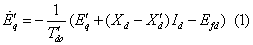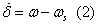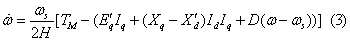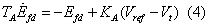Also ignoring the stator resistance of the synchronous machine, the algebraic equations governing stator can be written as: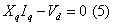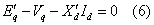In addition, the equations governing this network can be written as: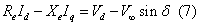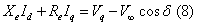Since small disturbances are considered in dynamic stability studies, the linearized system equations can be used.

In this paper, we assume that besides the variation in the system operating point, the transmission line reactance Xe also changes. Here the variation of Xe implies the variation in configuration and/ or structure of the system. Since Xe is not measurable in practice, it cannot be used as an input to fuzzy logic networks. As we know, the variation in transmission line reactance actually makes the reactive power production of generator (Q) change so that Xa can be substituted by Q. Therefore, we assume that in this system, the active power production of generator (P), generator terminal voltage (Vt), and even transmission line reactance (Xe) are variable and the other system parameters including the amplitude of infinite bus voltage are constant. Now ignoring the transmission line resistance (, i.e. Re=0), the following equation can be written from Figure 1: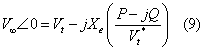Using eq. (9) and considering the definition,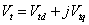we get: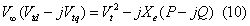Separating real and imaginary parts of eq. (10) and considering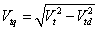, gives two following equations: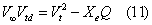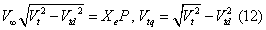Now with the knowledge of Vt (terminal voltage) and P (active power) as well as the infinite bus voltage and reactance Xe, we only have two unknowns in eqs. (11) and (12) which are Q and Vtd. Equations.(11) and (12) are nonlinear equations which their solution involves using a mathematical method for the calculation of Q and Vdt. We have used Broyden method  for solving above equations. The important feature of Broyden method is that it doesn’t require Jacobian matrix. In fact, it can be said that with the variation in system operating points, i.e. P, Vt, and the transmission line reactance Xe, the active power production of generator Q can be calculated from (11) and (12), and then P, Q and Vt are used as inputs to fuzzy logic network. The only remaining issue is that in eqs. (1) to (8), two variables, δ and θ, is needed which their computation is as follows.

Ignoring the stator resistance as well as the transmission line reactance, the active power production of generator is given by: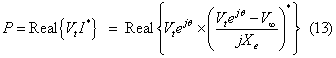Solving the equation above, we get: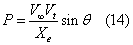Therefore, with the knowledge of P, Vt, Xeand also the infinite bus voltage, the angle of generator terminal voltage θ can be simply obtained by using eq. (14). Moreover, the angle of synchronous machine internal voltage δ can be calculated as follows: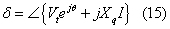Where,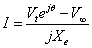#### Conventional Power System Stabilizers Design

As we know, currently the usage of conventional power system stabilizers (CPSS) is the most economic way for enhancing the power systems damping due to the low frequency oscillations occurrence. This stabilizer actually creates a damping torque in phase with generator speed variations. The CPSS input can be the speed deviation of a generator from synchronous speed, frequency variations of the system and/or accelerating torque designed by the phase compensation method [20-22]. The block diagram of such a PSS along with the linearized equations of a single machine system connected to an infinite bus presented in the previous section is given in Figure 2.

where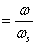The block diagram of a CPSS involving a two stages lead/lag compensator block, a washout block and an amplification factor KSTAB is shown in Figure 3.

The aim of lead/lag blocks in the model of a CPSS is cancellation of lagging caused by blocks following PSS which have been shown in Figure 2 with dashed lines as the transfer function GEP(s). Also it can be shown that ignoring constant K4, the transfer function GEP(s) is calculated as follows [20-22]: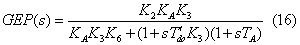As mentioned before, the aim of lead/lag blocks in a CPSS is cancellation of lagging caused by the transfer function GEP(s) during the low frequency oscillations of a synchronous machine. Ignoring the inherent damping of synchronous machine which implies D=0 in the machine model, the frequency of these oscillations is [20-22]: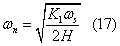Now if we denote the created phase angle by a block GEP(s) of frequency s=jωn with β, then considering a lead/lag block with m similar stages and also assuming time constants T1 and T2 for these blocks, these constants are given by: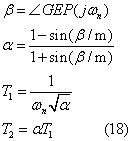As said, here we have used a two stages lead/lag block (m=2) in a CPSS. Now assuming a value for the damping coefficient ξ, the stabilizer gain factor (KSTAB) can be calculated as follows [20-22]: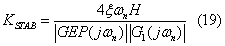Where G1(s) is the transfer function of a m stages lead/lag block which can be represented by: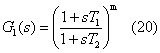Also, we have assumed the value of ξ=0.7 for all system operating points. Finally, considering the CPSS block involving a gain KSTAB and a two stages lead/lag block corresponding to T1 and T2 time constants, the linearized equations of the system can be written as the following state equations from Figure 2 (because of papers limitation, we ignore the computational details):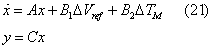where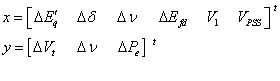in the expressions above, V1 and VPSS are two additional state variables which created by the CPSS block in the system and have been shown in Figure 3. Also A, B1, B2 and C are as: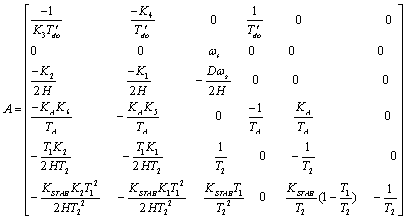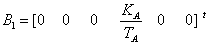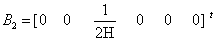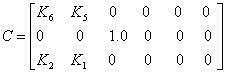Here we initially want to show which shape does the root locus of the system take with and without PSS (Figures 4 and 5).

#### Fuzzy Logic Network, Data Base rules and the Usage Method

Fuzzy logic controller structure

The conventional stabilizer is tuned for one operating point, but using fuzzy logic network and training with clustering method, it can be used for a wide range of the system operating points. Fuzzy systems are nonlinear and based on human knowledge. The core of these systems is a rules base which composes of fuzzy IF-THEN rules. A fuzzy IFTHEN rule is an if-then expression which some of its words specify by means of the fuzzy membership functions. As said, utilizing human experience in the form of formula is difficult. Fuzzy logic provides a simple means for such an application. As shown in Figure 6, the basic structure of a fuzzy logic controller includes three following parts .

1. Fuzzifiers- The inputs are read or measured and then we change the measured values to the form corresponding to the linguistic variable (the values proportional to membership function values).

2. Fuzzy inference engine-includes rules that relate the input membership functions to the output ones.

3. defuzzifiers- includes functions which transform the fuzzy output in the form of membership functions to a specified and acceptable point for the general output of the system [1,4,7,18].

There are three kinds of fuzzy systems:

1. Pure fuzzy system

2. Takagi-Sugeno-Kang fuzzy systems

3. Systems with fuzzifiers and defuzzifiers

The basic structure of fuzzy inference systems is a model which maps input properties to input membership functions, input membership functions to rules, rules to a set of output properties, output properties to output membership functions and ultimately output membership functions to a unique output value and/or a decision. Fuzzy inference is only used for modeling systems which their related rules were already determined by your interpretation of application. Figure. 6 represent the simple model of a fuzzy system. Sometimes in system modeling you cannot specify membership function by means of data investigation. Although the parameters associated with membership function can be arbitrarily determined, it is to be noted that the appropriate selection of these parameters would have a substantial effect on the system performance. In such cases, the design techniques of fuzzy systems can be used which one of them is the clustering method where an optimal tuning of the membership functions’ parameters is achieved by finding the class center and designating the membership functions appropriate to the class center [1,4,7].

Types of fuzzy system design methods are:

1. First order estimator

2. Second order estimator

3. Look-up table

5. Clustering method

6. Fuzzy C-means clustering analysis

Explanation of the final method, i.e. FCM clustering analysis, is the aim of this paper [13,14,18].

Fuzzy C-Means (FCM) clustering analysis

There isn’t a systematic procedure for specifying the number of fuzzy system rules in the previous methods. In the gradient descent method, the number of rules was fixed before training. In the table and estimator methods, the number of fuzzy sets is determined and then this number initially specifies the number of rules (Figure 7).

Clustering method includes an algorithm for selecting the number of rules . The input/output data sets divides into some clusters and a rule is expressed for each cluster.

First, a proper algorithm is selected for the limited number of input/output pairs, and then the nearest neighbour method is applied for clusters selection, and finally the optimum fuzzy system is designed for data fitting .

Assume an input/output pair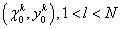where N is small. Our task is developing a fuzzy system that is able to match all N input/ output pairs with an arbitrary given accuracy. This requires that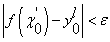,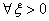To summarize, the training steps are given in the appendix. And now we assume that the trained fuzzy logic network of interest is ready

The shape of input membership functions after training is shown in Figure 8.

#### Simulation Results

In order to obtain the training data for fuzzy logic network, we assumed that the active power production of generator (P) is 0.5 to 1.1 times the rated active power production of generator. We also assumed that the amplitude of generator voltage is 0.95 to 1.05 times the nominal terminal voltage. The transmission line reactance was assumed constant. To produce training vectors, each time a random number with uniform distribution is independently selected for P and Vt in the range of associated variations and then solving eqs. (11) and (12) presented in section 2 gives the amount of reactive power production of generator (Q). Finally, we calculate the optimum values of stabilizer gain (KSTAB) and time constants T1 and T2 with the phase compensation method. Here we have used a stabilizer with a two stages lead/lag block for the random operating points. Therefore, a training pattern (vector) for fuzzy logic network actually includes P, Xe=cte, and V as inputs and KSTAB, T1 and T2 as outputs.

Using the method explained above, we generated 2000 training patterns and trained the fuzzy logic network with FCM clustering method. After some simulations and training, we investigated the network performance considering a neighboring radius of 0.5 for Gaussian membership functions. Test results show that Root Meansquared Error (RMSE) for 3 desirable outputs, KSTAB, T1 and T2 and the values obtained by trained fuzzy logic network is 0.7278,0.0138, and 0.0053, respectively which implies the ability of trained fuzzy logic network in good estimation of the PSS parameters. Estimations of the fuzzy logic network for 50 test data are shown in Figure 10.

As said earlier, one of the main problems with conventional power system stabilizers is that they are only tuned for only one system operating point. The optimum performance of these stabilizers requires retuning of these parameters as the system operating points change. To investigate this and also the advantages of online tuning of CPSS parameters using fuzzy logic network, we studied the performance of a single machine system connected to an infinite bus with applying two types of disturbance to the system. For the first disturbance, the variations in the generator terminal voltages (Vt) are plotted for a 0.1 p.u. step change in the generator reference excitation voltage (Vref). For the second disturbance, the variations in generator speed (ω) for a 0.5 p.u. step change in the machine input mechanical power is plotted. This is done for two operating points and we considered these three situations:

1. Single machine system without PSS

2. Single machine system with constant parameters PSS

3. Single machine system with PSS whose parameters retuned online for each operating point of the trained fuzzy logic network

The comparison for the first operating point is done among all 3 situations and for the second one, it is only done between (b) and (c). In the case of constant parameters PSS, the values of PSS parameters for both operating points were obtained considering the nominal values of the single machine system parameters and then the obtained values assumed constant for all system operating points. For the first operating point, the diagrams of the generator terminal voltage variations and generator speed variations are shown in Figures 11 and 12, respectively. For the second operating point, these diagrams are shown in Figures 13 and 14, respectively. These diagrams are associated with a random system operating point which is much different from the nominal operating point. As can be seen from these two figures, PSS with parameters tuned by FCM practically achieved damping of created oscillations, and also the system without PSS was unsuccessful in damping these oscillations and hence the system has become unstable.

Operating points are as:

• Operating point-1:

Active Power (P)= 0.6;

• Operating point-2: with 0.4 p.u. change in the transmitted power and increasing it to 1 p.u.

#### Conclusion

In this paper, an effective method as been presented for the online tuning of parameters of conventional power system stabilizers (CPSS) using fuzzy logic network (Fuzzy C-Means Clustering). The inputs are quantities like the active power production which are directly measurable, and hence after training network, the optimum stabilizer values corresponding to the current system operating point can be obtained. The required equations for designing CPSS with the phase compensation method have been completely explained. Considering some disturbances, the performance of the dynamic system equipped with PSS with parameters tuned by fuzzy logic network (Fuzzy C-means) was studied. The simulation results verified good performance of the designed PSS. Also FCM based PSS is able to create good damping over a wide range of the system operating points. More damping means the generator can be utilized stably at its maximum capacity, leading to economic saving of cost. Table 1 shows a comparison of stabilizers based on three networks, i.e. MLP, RBF and Fuzzy C-means.

Type RMSE
Fuzzy C-Means 0.0053
MLP 0.5168
RBF 0.7183

Table 1: The comparison of stabilizers for T2.

In this paper, a hybrid approach for tuning and placement of power system stabilizers (PSS) in multi-machine power systems is provided with the aim of reducing low-frequency oscillations (LFO) and improving power system dynamic stability with wide range of changes in system parameters and operating point of the system. PSS parameters are adjusted using Particle Swarm Optimization algorithm (PSO), and using Takagi–Sugeno (TS) fuzzy, optimal location for the PSS is determined. The employed fuzzy system has two inputs, the real part and the damping coefficient of network eigen values. The results of test on a grid four machine-two area sample, show optimal performance of the proposed method in improving system stability and reducing low-frequency oscillations of local and inter-area modes Figure 15.

It is clear from Table 1 that the neural network estimation has more error. Moreover, training time with clustering method was very low within 1 to 2 s, but training neural network has done in 39 iterations in a very large time.

#### Appendix

Case Study of the System:

XE=0.5, V=1, Tw=2, f=60, Tdo=9.6, xd=2.5, xpd=0.39, xq=2.1, H=3.2,

KA=400, TA=0.2, Ra=0, Re=0, Eb=1.05

The steps of Fuzzy C-Means network training are as follows.

Step 1: starting from input/output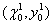we create a cluster with the center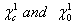and we put: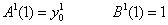Now we select a value for the radius, r.

Step 2: introducing the kth input/output pair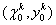, we have M clusters with centers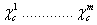. We calculate the distance of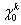from the center of these M clusters as: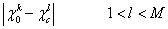and we take the lowest distance, i.e.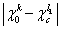a) if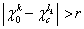, thenis a new class center and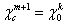We put: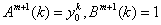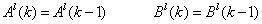b) if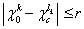, then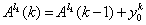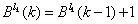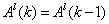c)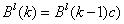Ifdoesn’t create a new cluster, then according to k input/ output pairs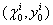, we get: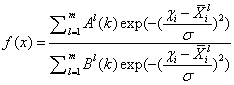And ifis a new cluster, M is substituted by M+1 in the new formula.

Step 3: step 2 with k=k+1

In the above relations,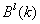is the number of input/output pairs in the lth cluster and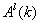is the sum of output values of its input/ output pairs. The radius r determines the degree of complexity of the designed fuzzy system.

Sinceandare calculated using recursive equations, a forgetfulness factor can be simply used which is useful for modeling systems with variable structures. Using this factor, we get: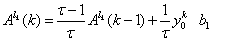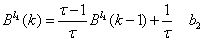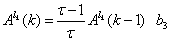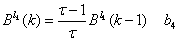Where τ is time constant of the decreasing exponential function. In practice,must have a threshold so that this cluster eliminates ifgoes below this threshold.

#### References

Select your language of interest to view the total content in your interested language

### Article Usage

• Total views: 14028
• [From(publication date):
June-2015 - Feb 25, 2020]
• Breakdown by view type
• HTML page views : 10102Can't read the image? click here to refresh